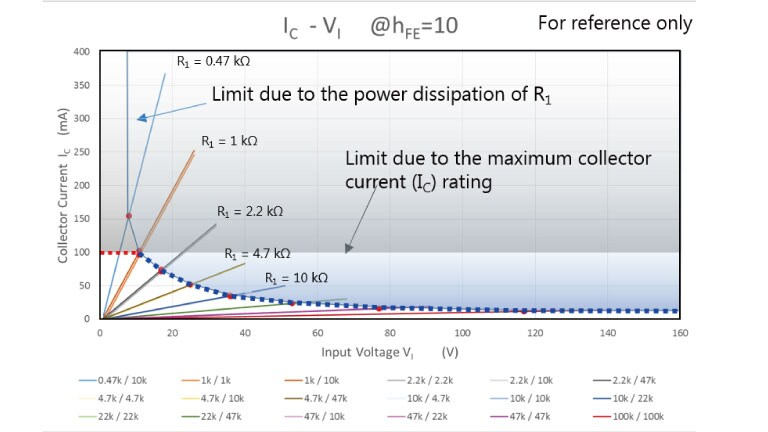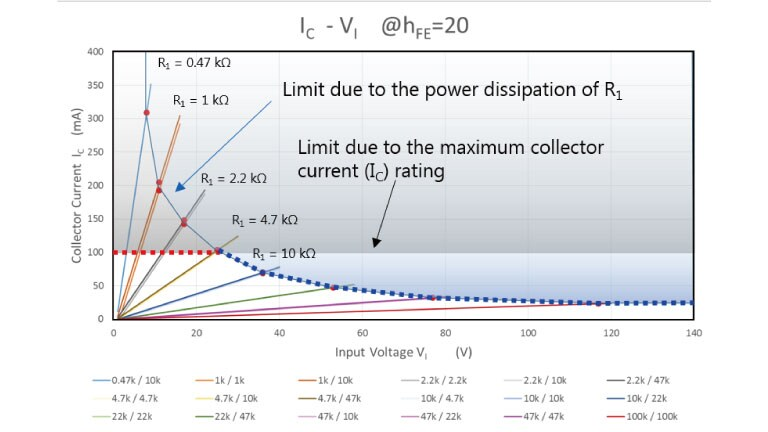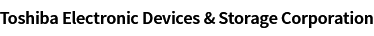### Cross Reference Search

About information presented in this cross reference

The information presented in this cross reference is based on TOSHIBA's selection criteria and should be treated as a suggestion only. Please carefully review the latest versions of all relevant information on the TOSHIBA products, including without limitation data sheets and validate all operating parameters of the TOSHIBA products to ensure that the suggested TOSHIBA products are truly compatible with your design and application.
Please note that this cross reference is based on TOSHIBA's estimate of compatibility with other manufacturers' products, based on other manufacturers' published data, at the time the data was collected.
TOSHIBA is not responsible for any incorrect or incomplete information. Information is subject to change at any time without notice.

### Stock Check & Purchase

Select Product Categories

Select Application

Find everything you need for your next product design. Simply select an application and click through to the block diagram to discover our semiconductor solutions.

New Products / News

Design & Development

Knowledge

# What is the maximum voltage that can be applied to the base of a bias resistor built-in transistor (BRT)? (How many watts is the allowable power dissipation of the built-in resistors?)

You do not need to consider the maximum allowable base voltage for typical applications (in which input voltage (VI) is lower than 10 V), but care is required when VI is higher than 10 V.
The maximum input voltage (VI) is mainly determined by the following factors:
1.    Value of the series base resistor (R1) (i.e., the power dissipated by R1)
2.    Maximum collector current (IC)
The maximum input voltage varies with the usage conditions of a BRT (e.g., hFE), but it is generally determined by the collector current when the R1 value is small and by the R1 value when it is large.

1. Relationship between the value of the series base resistor (R1) and the input voltage (VI)
The allowable power dissipation of the built-in resistors of a BRT is 1/8 W. Let the base-emitter voltage of the internal transistor in the “on” state be Vbe. Then, the current flowing through R1 (IB) is expressed as follows. For the sake of simplicity, let Vbe = 0.7 V.
IB ＝ ( VI – Vbe ) / R1 = ( VI – 0.7 ) / R1
The power dissipation of R1 due to IB must not exceed 1/8 W. Hence:
1/8 W > R1 * { ( VI – 0.7 ) / R1 }^2
> ( VI – 0.7 ) ^2 / R1
VI < √( R1 / 8 ) + 0.7

2. Relationship between the maximum collector current (IC(max)) and the input voltage (VI)
The maximum collector current (IC(max)) is specified as an absolute maximum rating, which must not be exceeded even instantaneously.
Since the BRT operates in the saturation region, its hFE is in the range of 10 to 20. The current flowing to the base (b) of the internal transistor (Ib) can be calculated by subtracting the current flowing to R2 (IR2) from the IB value calculated above:
Ib = IB – IR2 = ( VI – Vbe ) / R1 – Vbe / R2 = ( VI – 0.7 ) / R1 – 0.7 / R2
Since the collector current (IC) is equal to Ib times hFE, the following equation must be satisfied:
IC (max) > IC = hFE * IB = hFE * {( VI – 0.7 ) / R1 – 0.7 / R2 }
VI < R1 * IC(max) / hFE +( R1 + R2 ) * 0.7 / R2
The lower of the VI values calculated based on the above two factors is the maximum allowable base voltage.
1. Value of the series base resistor (R1)
VI < √( R1 / 8 ) + 0.7
2. Maximum collector current (IC(max))
VI < R1 * IC (max) / hFE +( R1 + R2 ) * 0.7 / R2
Figure 2 and Figure 3 show the relationships between input voltage and collector current at different resistor values.
Although the maximum input voltage and its limiting factors depend on the hFE (IC/IB) of the BRT, the following are true:

• When the R1 value is small: The maximum input voltage is determined by the absolute maximum collector current specified in the datasheet.
• When the R1 value is large: The maximum input voltage is determined by the power dissipation of R1.Figure 2 Input voltage vs. collector current (when hFE = 10)Figure 3 Input voltage vs. collector current (when hFE = 20)
A new window will open
Site Map

Semiconductor Products

Storage Products

Other Products

Company